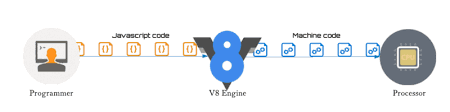## 编译原理

• 词法分析(laxical Analysis) 词法分析的意思就是，将代码块切分成最小的单位。这些最小单位称为token。 比如 var a = 2;可以切分成var,a,=,2。
• 语法分析(Syntactic Analysis) 将词法单元转换成一个有层级，代表程序语法结构的树，这就是我们经常说的AST，抽象语法树。

``````function add (a, b) {
return a + b
}
``````

AST可是所有编译器以及转换器的基础核心，我们常用的babel转码过程就是先将ES6的代码编成AST，然后转换成ES5的AST，最后由这个AST还原出ES5代码。有兴趣的可以看这篇文章，这篇文章是将LISP-style代码的转成C-style代码，不过原理都一样。

• 代码生成(Code Genaration)

V8引擎的编译过程基本就是上面这个过程，但是它多了一步生成字节码的过程。 首先用解析器生成AST，然后用解释器Ignition根据语法树生成字节码，最后再用TurboFan将字节码生成机器指令码。

## V8 为什么那么快

1、脚本流(script streaming)

2、字节码缓存

3、内联

``````function add(a, b) {
return a + b;
}
function calculateTwoPlusFive() {
var sum;
for (var i = 0; i <= 1000000000; i++) {
}
}
var start = new Date();
calculateTwoPlusFive();
var end = new Date();
var timeTaken = end.valueOf() - start.valueOf();
console.log("Took " + timeTaken + "ms");
``````

``````function add(a, b) {
return a + b;
}
function calculateTwoPlusFive() {
var sum;
for (var i=0;i<=1000000000;i++){
sum = 2 + 5;
}
}
var start = new Date();
calculateTwoPlusFive();
var end = new Date();
var timeTaken = end.valueOf() - start.valueOf();
console.log("Took " + timeTaken + "ms");复制代码
``````

4、隐藏类

``````function Person(name, age) {
this.name = name;
this.age = age;
}

var daisy = new Person("daisy", 32);
var alice = new Person("alice", 20);

daisy.email = "daisy@qq.com";
daisy.job = "engineer";

alice.job = "engineer";
alice.email = "alice@qq.com";
``````

5、热点函数会被直接编译成机器码

v8在运行的时候，会采集JS代码运行数据。当发现某个函数被频繁调用，那么就会将它标记成热点函数，并且认为他是一个类型稳定的函数。这时候会将它生成更为高效的机器码。

``````function add(a, b){
return a + b
}
for(var i=0; i<10000; ++i){
}
// 最后却传了string，会退回到字节码，会使得性能受损
``````

``````// 片段 1
var person = {
return a + b;
} };
obj.name = 'li';

// 片段 2
var person = {
return a + b;}
name: 'li'
};
``````

1. 脚本流

2.字节码缓存

3.内联

4.隐藏类

5.热点函数编译成机器码